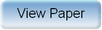Ninth International Geostatistics Congress, Oslo, Norway
June 11 – 15, 2012Session: Posters Abstract No.: P-007 Title: Validity range of the discrete Gaussian change-of-support model and its variant Author(s): Jean-Paul Chilès, MINES ParisTech (FR) Abstract: The discrete Gaussian model is a very popular change-of-support model. It is designed for a stationary random function Z(x) that is regarded as the transform of a stationary random function Y(x) with Gaussian marginal. The average value Z(v) in a block v is considered as another transform of some Gaussian random variable Yv (which is not the average Y(v) of Y(x) in v). The basic assumption of the discrete Gaussian model is that the bivariate distribution of Yv and Y(u), where u is a random point in v, is Gaussian. Extensions enable the estimation of a block by disjunctive kriging or in a multivariate Gaussian framework. While the initial model (DGM1) was first formulated by G. Matheron, a variant (DGM2) has been proposed by X. Emery. In this variant the Gaussian random variable Yv associated with the average grade Z(v) of block v is Y(v) / r where r is the variance of Y(v). This induces large simplifications in the use of the model, and more specifically in its multivariate Gaussian extension. Both models are approximations but DGM2 works under the additional assumption that the bivariate distribution of Y(u) and Y(u') is Gaussian, where u and u' are two independent random points in v. The variant DGM2 is therefore based on a priori stronger assumptions than the initial model DGM1. Validity checks have been given for either model but under different conditions (Matheron, Emery, Cressie). We compare the two models with the exact model obtained by means of simulations in order to define their respective validity ranges and determine when DGM2 works better than, or as well as, DGM1.Produced by X-CD Technologies Inc.